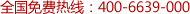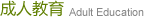# 2012年成人高考数学模拟试题（文史财经类）

2012年成人高考数学模拟试题（文史财经类）

一、选择题（17小题，每小题5分共85分）
1、设集合A={0，3}，B={0，3，4}，C={1，2，3}，则(B∪C)∩A=__________
A、{0,1,2,3,4}      B、空集       C、{0,3}       D、{0}
2、非零向量a∥b的充要条件___________________
A、 a=b    B、 a=-b     C、 a=±b     D、 存在非零实数k,a=kb
3、二次函数 y=x2+4x+1的最小值是_________________
A、  1    B、  -3      C、  3      D、  -4
4、在等差数列{an}中,已知a1=-,a6=1 则__________
A、 a3=0    B、 a=0    C、 a=0      D、 各项都不为零
5、函数y=x3+2sinx__________
A、 奇函数  B、 偶函数  C、 非奇非偶函数D、 既是奇函数又是偶函数
6、已知抛物线y=x2在点x=2处的切线的斜率为___________
A、  2    B、  3    C、  1    D、  4
7、直线L与直线3x-2y+1=0垂直，则1的斜率为__________
A、3/2    B  -3/2   C、 2/3    D、 -2/3
8、已知=（3，2）=(-4,6),则=____________
A、4    B、 0     C、-4    D、5
9、双曲线-=1的焦距是___________
A、4    B、   C、2    D、8
10、从13名学生中选出2人担任正副班长，不同的选举结果共有（）
A、26   B、78      C、156      D、169
11、若f(x+1)=x2+2x,则f(x)=_________
A、x2-1  B、x2+2x+1   C、x2+2x  D、 x2+1
12、设tanx=,且cosx<0,则cosx的值是_______
A、-   B、     C、     D、-
13、已知向量a,b满足=4，=3,<a,b>=300 则ab=
A、   B、6   C、6      D、12
14、函数y=sin(3x+)的最小正周期________
A、3   B、      C、   D、
15、直线2x-y+7=0与圆（x-1）2+(y+1)2=20
A、相离   B、相切  C、相交但直线不过圆心   D、相交且直线过圆心
16、已知二次函数y=x2+ax-2的对称轴方程为x=1,则函数的顶点坐标______
A.（1，-3）   B.（1，-1）   C.（1，0）      D（-1，-3）
17、椭圆9x2+16y2=144的焦距为_______
A、10  B、5  C、2    D、14

1、函数y=㏒2(6-5x-x2)的定义域____________
2、不等式<8的解集是_______________
3、已知A（-2，1） B、（2，5），则线段AB的垂直平分线的方程是____________
4、某篮球队参加全国甲级联赛，任选该队参赛的10场比赛，其得分情况如下：
99，104，87，88，96，94，100，92，108，110，则该队得分的样本方差为______

1、求函数y=x4-2x2+5在区间[-2，2]上最大值和最小值  （10分）

2、设{an}为等差数列，Sn表示它的前n项和，已知对任何正整数n均有Sn=+n, 求数列{an}的公差d和首项a1    （10分）

3、已知直线在X轴上的截距为-1，在Y轴上的截距为1，对抛物线y=x2+bx+c的顶点坐标（2，-8），求直线和抛物线两个交点横坐标的平方和。（12分）

4、设点P是双曲线3x2-y2=3右支上一点，F1、F2、分别是双曲线的左、右焦点，△PF1F2周长为10，求tan<PF1F2的值。 （13分）

2011年成人高考数学模拟试题答案：
一、选择题（17小题，每题5分，共85分）
1、C   2、D   3、B   4、B   5、A   6、D  7、D  8、B  9、C  10、C  11、A
12、D  13、B  14、C  15、B  16、A  17、C

1、{x}    2、{x}    3、y+x-3=0   4、56.16

1、解　y＇＝４-4x＝４x（x2-1）
令y＇＝０　　得出x1=0    x2=1    x3=-1
f(0)=5,   f(1)=4    f(-1)=4
f(-2)=13            f(2)=13

２、解：　　　　a1=s1=

S2==3+a2       解得a2=6
d=a2-a1=3

3、因为抛物线的顶点坐标（2，-8）
-=2           解得：b=-4,   c=-4
则抛物线方程y=x2-4x-4
y=x+1
y=x2-4x-4     推出:x2-5x-5=0的两个根x1和x2，
那么：x1+x2=5,   x1x2=-5
所以：  + = (x1+x2)2-2x1x2=35
4、由双曲线的方程可知：a2=1,a=1   b2=3,  c2=4,c=2

-=2，=2C=4

Cos<PF1F2==
Tan<PF1F2==